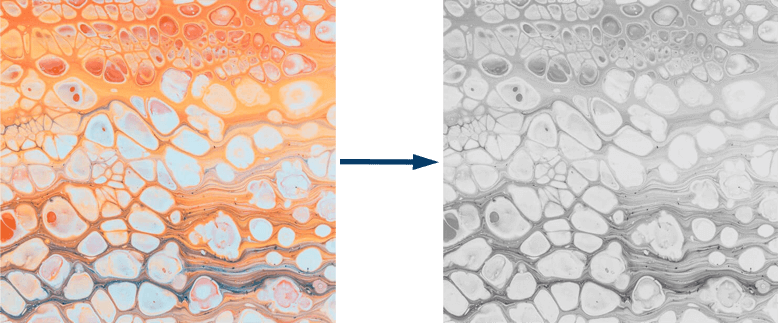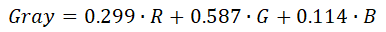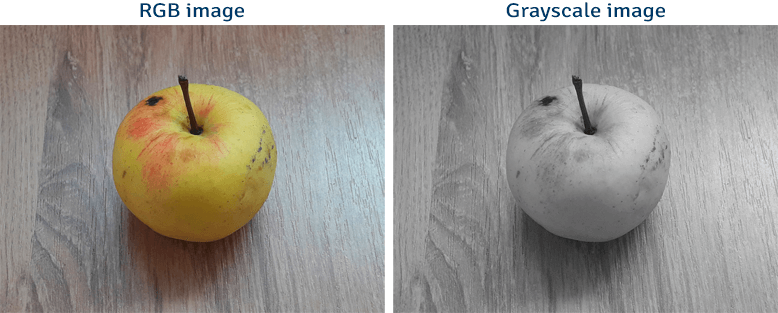# Convert RGB Image to Grayscale Image using OpenCV

During image processing RGB images are often converted to grayscale images because smaller amount of data allows to perform more complex image processing operations faster.

This tutorial provides example how to convert RGB image to grayscale image using OpenCV.

OpenCV provides `cvtColor` function that allows to convert an image from one color space to another. This function accepts color conversion code. `BGR2GRAY` code is used to convert RGB image to grayscale image. Note that, OpenCV loads an image where the order of the color channels is Blue, Green, Red (BGR) instead of RGB.

OpenCV uses weighted method, also known as luminosity method for RGB to grayscale conversion. Grayscale value is calculated as weighted sum of the R, G, and B colour components. The formula is:``````import cv2

grayImg = cv2.cvtColor(img, cv2.COLOR_BGR2GRAY)

cv2.imshow('RGB image', img)
cv2.imshow('Grayscale image', grayImg)
cv2.waitKey(0)
cv2.destroyAllWindows()``````
``````#include <opencv2/opencv.hpp>

using namespace cv;

int main()
{
Mat grayImg;
cvtColor(img, grayImg, COLOR_BGR2GRAY);

imshow("RGB image", img);
imshow("Grayscale image", grayImg);
waitKey(0);
destroyAllWindows();

return 0;
}``````
``````package app;

import org.opencv.core.Core;
import org.opencv.core.Mat;
import org.opencv.highgui.HighGui;
import org.opencv.imgcodecs.Imgcodecs;
import org.opencv.imgproc.Imgproc;

public class Main
{

public static void main(String[] args)
{
Mat grayImg = new Mat();
Imgproc.cvtColor(img, grayImg, Imgproc.COLOR_BGR2GRAY);

HighGui.imshow("RGB image", img);
HighGui.imshow("Grayscale image", grayImg);
HighGui.waitKey(0);
HighGui.destroyAllWindows();

System.exit(0);
}
}``````

Result: